Alert indicator I converted MQL4 to MQL5 doesn't work at all.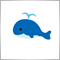80

Hello.

This MQL4 alert indicator gives alerts everytime the price touches the horizontal lines or trendlines you drew.

I converted it to MQL5, and it doesn't work at all.

Can anyone help me with this problem, please?    Thank you in advance.

//MQL4

#define PriceMAX 999999
#property indicator_chart_window

extern bool AlertMode = true;
extern bool SoundMode = true;
extern int SoundInterval = 30;
extern bool Check_HorizontalLine = true;
extern bool Check_TrendLine = true;

int start()
{
int obj_total = ObjectsTotal();
string name;
for (int i = 0; i < obj_total; i++)
{
name = ObjectName(i);
if (ObjectType(name) != OBJ_HLINE &&
ObjectType(name) != OBJ_TREND)
continue;
if (!Check_HorizontalLine &&
ObjectType(name) == OBJ_HLINE)
continue;
if (!Check_TrendLine &&
ObjectType(name) == OBJ_TREND)
continue;

double _price, _price1;
if (ObjectType(name) == OBJ_HLINE)
{
_price = ObjectGet(name, OBJPROP_PRICE1);
_price1 = _price;
}
if (ObjectType(name) == OBJ_TREND)
{
_price = ObjectGetValueByShift(name, 0);
_price1 = ObjectGetValueByShift(name, 1);
}

//Print(i, "Object name for object #", i, " is"
//       + name, " ", _price, " C = ", Close,
//                            " O = ", Open);

if ((_price > Open || _price1 > Close) &&
Close >= _price)
if ((_price < Open || _price1 < Close) &&
Close <= _price)
}
return(0);
}

void MyAlert(string name, string dir, double _price){
static double LastUpPrice = 0;
static double LastDownPrice = PriceMAX;
static datetime LastAlertTime = 0;

if (LastAlertTime != Time)
{
LastUpPrice = 0;
LastDownPrice = PriceMAX;
}

if (dir == "up" && _price > LastUpPrice)
{
LastUpPrice = _price;
+DoubleToStr(Close, Digits)+" over "
+DoubleToStr(_price, Digits)+" ["+name+"]");

}
else if (dir == "down" && _price < LastDownPrice)
{
LastDownPrice = _price;
+DoubleToStr(Close, Digits)+" under "
+DoubleToStr(_price, Digits)+" ["+name+"]");

}

if (SoundMode)
{
static datetime LastSoundTime = 0;
if (TimeLocal() < LastSoundTime+SoundInterval)
return;

LastSoundTime = TimeLocal();
if (dir == "up")
{
//PlaySound("expert");
PlaySound(StringSubstr(Symbol(), 0, 6)+".wav");
//EURUSD.wav, AUDJPY.wav...
}
else if (dir == "down")
{
//PlaySound("expert");
PlaySound(StringSubstr(Symbol(), 0, 6)+".wav");
}
}
}
//MQL5 , This doesn't work.

#define PriceMAX 999999
#property indicator_chart_window

input bool AlertMode = true;
input bool SoundMode = true;
input int  SoundInterval = 30;
input bool Check_HorizontalLine = true;
input bool Check_TrendLine = true;

int OnCalculate(const int rates_total,
const int prev_calculated,
const datetime &time[],
const double &open[],
const double &high[],
const double &low[],
const double &close[],
const long &tick_volume[],
const long &volume[],
{

int obj_total = ObjectsTotal(0, 0, -1);
string name;

for (int i = 0; i < obj_total; i++)
{
name = ObjectName(0, i, 0, -1);

if(ObjectGetInteger(0, name, OBJPROP_TYPE) != OBJ_HLINE &&
ObjectGetInteger(0, name, OBJPROP_TYPE) != OBJ_TREND)
continue;

if (!Check_HorizontalLine &&
ObjectGetInteger(0, name, OBJPROP_TYPE) == OBJ_HLINE)
continue;

if (!Check_TrendLine &&
ObjectGetInteger(0, name, OBJPROP_TYPE) == OBJ_TREND)
continue;

double _price, _price1;

double Close[];
ArraySetAsSeries(Close, true);
CopyClose(_Symbol, PERIOD_CURRENT, TimeCurrent(), 2, Close);

datetime Close_time[];
ArraySetAsSeries(Close_time, true);
CopyTime(_Symbol, PERIOD_CURRENT, TimeCurrent(), 2, Close_time);

double Open[];
ArraySetAsSeries(Open, true);
CopyOpen(_Symbol, PERIOD_CURRENT, TimeCurrent(), 2, Open);

if (ObjectGetInteger(0, name, OBJPROP_TYPE) == OBJ_HLINE)
{
_price = ObjectGetDouble(0, name, OBJPROP_PRICE);
_price1 = _price;
}

if (ObjectGetInteger(0, name, OBJPROP_TYPE) == OBJ_TREND)
{
_price  = ObjectGetValueByTime(0, name, Close_time);
_price1 = ObjectGetValueByTime(0, name, Close_time);
}

if ((_price > Open || _price1 > Close) &&
Close >= _price);

if ((_price < Open || _price1 < Close) &&
Close <= _price);
}
return(rates_total);
}

void MyAlert(string name, string dir, double _price){
static double LastUpPrice = 0;
static double LastDownPrice = PriceMAX;
static datetime LastAlertTime = 0;

datetime Time[];
ArraySetAsSeries(Time, true);
CopyTime(_Symbol, PERIOD_CURRENT, TimeCurrent(), 2, Time);

double Close[];
ArraySetAsSeries(Close, true);
CopyClose(_Symbol, PERIOD_CURRENT, TimeCurrent(), 2, Close);

if (LastAlertTime != Time)
{
LastUpPrice = 0;
LastDownPrice = PriceMAX;
}

if (dir == "up" && _price > LastUpPrice)
{
LastUpPrice = _price;

+DoubleToString(Close, _Digits)+" over "
+DoubleToString(_price, _Digits)+" ["+name+"]");
}

else if (dir == "down" && _price < LastDownPrice)
{
LastDownPrice = _price;
+DoubleToString(Close, _Digits)+" under "
+DoubleToString(_price, _Digits)+" ["+name+"]");
}

if (SoundMode)
{
static datetime LastSoundTime = 0;
if (TimeLocal() < LastSoundTime+SoundInterval)
return;

LastSoundTime = TimeLocal();
if (dir == "up")
{
//PlaySound("expert");
PlaySound(StringSubstr(_Symbol, 0, 6)+".wav");
//EURUSD.wav, AUDJPY.wav...
}

else if (dir == "down")
{
//PlaySound("expert");
PlaySound(StringSubstr(_Symbol, 0, 6)+".wav");
}
}
}19852

1. yogureee: it doesn't work at all.
"Doesn't work" is meaningless - just like saying the car doesn't work. Doesn't start, won't go in gear, no electrical, missing the key, flat tires - meaningless. We can't see your broken code. There are no mind readers here and our crystal balls are cracked.

2.       double Close[];
ArraySetAsSeries(Close, true);
CopyClose(_Symbol, PERIOD_CURRENT, TimeCurrent(), 2, Close);

datetime Close_time[];
ArraySetAsSeries(Close_time, true);
CopyTime(_Symbol, PERIOD_CURRENT, TimeCurrent(), 2, Close_time);

double Open[];
ArraySetAsSeries(Open, true);
CopyOpen(_Symbol, PERIOD_CURRENT, TimeCurrent(), 2, Open);
Why are you doing this when you already have arrays open[], close[], and time[]?

3. Use the debugger or print out your variables, including and find out why.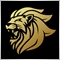Moderator
12471

If you use the latest build you should be able to use the same MQL4 code thanks to the newly added functions.80

whroeder1:
1. "Doesn't work" is meaningless - just like saying the car doesn't work. Doesn't start, won't go in gear, no electrical, missing the key, flat tires - meaningless. We can't see your broken code. There are no mind readers here and our crystal balls are cracked.

2. Why are you doing this when you already have arrays open[], close[], and time[]?

3. Use the debugger or print out your variables, including and find out why.

Thank you for taking time reading my wrong code and for your advice.

I will post here again when I find out the problems and how I should code it.

For now, it is difficult to see whether the code is redundant or not.

I use " Print ("A = ", A); " for both MT4 and MT5, and it is very helpful to see how the code works.

I will try Debug.80

Marco vd Heijden:
If you use the latest build you should be able to use the same MQL4 code thanks to the newly added functions.

Thank you for the information.

I use the latest build,  Version: 5.00  build 1816.

I'm afraid I can't find the newly added functions that enable MQL4 code be available in the MT5 MetaEditor.

How can I find them or articles about it?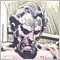3625

yogureee:

Thank you for the information.

I use the latest build,  Version: 5.00  build 1816.

I'm afraid I can't find the newly added functions that enable MQL4 code be available in the MT5 MetaEditor.

How can I find them or articles about it?

By "latest build" I think he meant 1845, which is in Beta. See the release statement:

https://www.mql5.com/en/forum/254082

Search for "MQL5: New functions: iTime, iOpen, iHigh, iLow, iClose, iVolume, iBars, iBarShift, iLowest, iHighest, iRealVolume, iTickVolume, iSpread."80

Anthony Garot:

By "latest build" I think he meant 1845, which is in Beta. See the release statement:

https://www.mql5.com/en/forum/254082

Search for "MQL5: New functions: iTime, iOpen, iHigh, iLow, iClose, iVolume, iBars, iBarShift, iLowest, iHighest, iRealVolume, iTickVolume, iSpread."

Thank you again for your information.

I will install the latest build on this weekend, and restart my converting when the stable version is released.

I'm looking forward to other new features too.80

We already have  ".......const double &close[]........ "  as formal parameters of  "int OnCalculate()" ,

so I don't need  "double Close[]......." anymore.   These are redundant.

I should use "close", and wrote like this:

ArraySetAsSeries(close, true);

CopyClose(_Symbol, PERIOD_CURRENT, TimeCurrent(), 2, close);

But I got an error.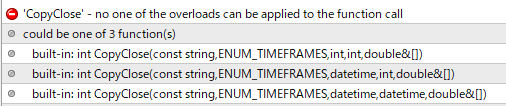I tried these 3 codes above.   Still error.

I read:     https://www.mql5.com/en/forum/1316         I still don't know what to do.

Converting is too difficult.

Instead, I modified the MQL4 alert indicator to MQL5 one which works but the codes are not good at all.

#property indicator_chart_window

input int  SoundInterval = 30;
input bool Check_HorizontalLine = true;
input bool Check_TrendLine = true;
bool AlertFlag = false;

int OnCalculate(const int rates_total,
const int prev_calculated,
const datetime &time[],
const double &open[],
const double &high[],
const double &low[],
const double &close[],
const long &tick_volume[],
const long &volume[],
{

int obj_total = ObjectsTotal(0, 0, -1);
//Print("obj_total: ", obj_total);

string name;

for (int i = 0; i < obj_total; i++)
{
name = ObjectName(0, i, 0, -1);

if(ObjectGetInteger(0, name, OBJPROP_TYPE) != OBJ_HLINE &&
ObjectGetInteger(0, name, OBJPROP_TYPE) != OBJ_TREND)
continue;

if (!Check_HorizontalLine &&
ObjectGetInteger(0, name, OBJPROP_TYPE) == OBJ_HLINE)
continue;

if (!Check_TrendLine &&
ObjectGetInteger(0, name, OBJPROP_TYPE) == OBJ_TREND)
continue;

double HorizontallinePrice, TrendlinePrice;

double Close[];
ArraySetAsSeries(Close, true);
CopyClose(_Symbol, PERIOD_CURRENT, TimeCurrent(), 2, Close);
//Print("Close = ", Close);

datetime Close_time[];
ArraySetAsSeries(Close_time, true);
CopyTime(_Symbol, PERIOD_CURRENT, TimeCurrent(), 2, Close_time);

double Open[];
ArraySetAsSeries(Open, true);
CopyOpen(_Symbol, PERIOD_CURRENT, TimeCurrent(), 2, Open);

if (ObjectGetInteger(0, name, OBJPROP_TYPE) == OBJ_HLINE)
{
HorizontallinePrice = ObjectGetDouble(0, name, OBJPROP_PRICE);
//Print("horizontalline_price =  ", HorizontallinePrice);
}

if (ObjectGetInteger(0, name, OBJPROP_TYPE) == OBJ_TREND)
{
TrendlinePrice  = ObjectGetValueByTime(0, name, Close_time);
//Print("trendline_price =  ", TrendlinePrice);
}

if((HorizontallinePrice > Open) && (HorizontallinePrice <= Close))
{
{
+DoubleToString(Close, _Digits)+" over "
+DoubleToString(HorizontallinePrice, _Digits)+" ["+name+"]");

}
}

else if((TrendlinePrice > Open) && (TrendlinePrice <= Close))
{
{
+DoubleToString(Close, _Digits)+" over "
+DoubleToString(TrendlinePrice, _Digits)+" ["+name+"]");

}
}

else if((HorizontallinePrice < Open) && (HorizontallinePrice >= Close))
{
{
+DoubleToString(Close, _Digits)+" under "
+DoubleToString(HorizontallinePrice, _Digits)+" ["+name+"]");
}
}

else if((TrendlinePrice < Open) && (TrendlinePrice >= Close))
{
{
+DoubleToString(Close, _Digits)+" under "
+DoubleToString(TrendlinePrice, _Digits)+" ["+name+"]");
}
}

else
{
}
}
return(rates_total);
}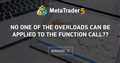no one of the overloads can be applied to the function call??
• 2010.07.06
• www.mql5.com
in mql4, i can use iTime to get bar opentime , in mql5 , i use below sentence CopyTime(Symbol(),PERIOD_CURRENT,1,1,from); but i get compile...Moderator
12471

When you open a demo account on MetaQuotes Demo Server then your terminal will shortly update to 1846 and you can omit copyclose and use the regulat iClose iOpen iHigh iLow and etc.

You can see these functions here: https://www.mql5.com/en/forum/254082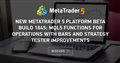New MetaTrader 5 Platform beta build 1845: MQL5 functions for operations with bars and Strategy Tester improvements
• 2018.06.08
• www.mql5.com
New MetaTrader 5 Platform beta build 1845: MQL5 functions for operations with bars and Strategy Tester improvements The new beta version of the upd...80

Marco vd Heijden:

When you open a demo account on MetaQuotes Demo Server then your terminal will shortly update to 1846 and you can omit copyclose and use the regulat iClose iOpen iHigh iLow and etc.

You can see these functions here: https://www.mql5.com/en/forum/254082

Thank you for the information.

I was able to get 1847.

Now I can forget about CopyClose(), and I will try iClose .... later.80

I used iTime(), iOpen(), iClose().    They are great.
ArraySetAsSeries(close, true);

iClose(_Symbol, PERIOD_CURRENT, 0);   // current bar

My codes got better.  I appreciate MT5, MQL5 experts who gave me advice and information here.

#property copyright "Copyright 2018, MetaQuotes Software Corp."
#property version   "1.00"
#property indicator_chart_window

input int  SoundInterval = 30;
input bool Check_HorizontalLine = true;
input bool Check_TrendLine = true;
bool AlertFlag = false;

int OnCalculate(const int rates_total,
const int prev_calculated,
const datetime &time[],
const double &open[],
const double &high[],
const double &low[],
const double &close[],
const long &tick_volume[],
const long &volume[],
{

int obj_total = ObjectsTotal(0, 0, -1);
Print("obj_total = ", obj_total);

string name;

for (int i = 0; i < obj_total; i++)
{
name = ObjectName(0, i, 0, -1);

if(ObjectGetInteger(0, name, OBJPROP_TYPE) != OBJ_HLINE &&
ObjectGetInteger(0, name, OBJPROP_TYPE) != OBJ_TREND)
continue;

if (!Check_HorizontalLine &&
ObjectGetInteger(0, name, OBJPROP_TYPE) == OBJ_HLINE)
continue;

if (!Check_TrendLine &&
ObjectGetInteger(0, name, OBJPROP_TYPE) == OBJ_TREND)
continue;

double HorizontallinePrice, TrendlinePrice;

ArraySetAsSeries(time, true);
iTime(_Symbol, PERIOD_CURRENT, 0);
Print("time = ", time);

ArraySetAsSeries(open, true);
iOpen(_Symbol, PERIOD_CURRENT, 0);
Print("open = ", open);

ArraySetAsSeries(close, true);
iClose(_Symbol, PERIOD_CURRENT, 0);
Print("close = ", close);

if (ObjectGetInteger(0, name, OBJPROP_TYPE) == OBJ_HLINE)
{
HorizontallinePrice = ObjectGetDouble(0, name, OBJPROP_PRICE);
Print("horizontalline_price =  ", HorizontallinePrice);
}

if (ObjectGetInteger(0, name, OBJPROP_TYPE) == OBJ_TREND)
{
TrendlinePrice  = ObjectGetValueByTime(0, name, time);
Print("trendline_price =  ", TrendlinePrice);
}

if((HorizontallinePrice > open) && (HorizontallinePrice <= close))
{
{
+DoubleToString(close, _Digits)+" over "
+DoubleToString(HorizontallinePrice, _Digits)+" ["+name+"]");

}
}

else if((TrendlinePrice > open) && (TrendlinePrice <= close))
{
{
+DoubleToString(close, _Digits)+" over "
+DoubleToString(TrendlinePrice, _Digits)+" ["+name+"]");

}
}

else if((HorizontallinePrice < open) && (HorizontallinePrice >= close))
{
{
+DoubleToString(close, _Digits)+" under "
+DoubleToString(HorizontallinePrice, _Digits)+" ["+name+"]");
}
}

else if((TrendlinePrice < open) && (TrendlinePrice >= close))
{
{
+DoubleToString(close, _Digits)+" under "
+DoubleToString(TrendlinePrice, _Digits)+" ["+name+"]");